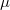﻿

### Frame measures for infinitely many measures

#### Abstract

For every frame spectral measure$\mu$, there exists a discrete measure$\nu$ as a frame measure. If$\mu$ is not a frame spectral measure, then there is not any general statement about the existence of frame measures$\nu$ for$\mu$. This motivated us to examine Bessel and frame measures. We construct infinitely many measures$\mu$ which admit frame measures$\nu$, and we show that there exist infinitely many frame spectral measures$\mu$ such that besides having a discrete frame measure, they admit continuous frame measures too.

DOI Code: 10.1285/i15900932v40n1p115

Keywords: Fourier frame; Plancherel theorem; spectral measure; frame measure; Bessel measure

Full Text: PDF